Look around you. Everything you can see is made of only three kinds of particles, in various configurations.

The seemingly infinite variation in color, texture, reactivity, taste, et cetera, in the objects in and around you, is all a result of the particular way that protons, neutrons and electrons come together to form those objects.

Equally amazing is that interactions among these three particles happens through one or more of only four mechanisms. That is, there are only four distinct types of forces that are responsible for all interactions among the lumpy sea of protons, neutrons and electrons that comprise our world.

Here are the four known methods through which particles can interact with one another; i.e. the four fundamental forces:

Strong nuclear force

Weak

nuclear force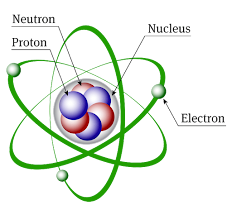Particles can only interact via the Strong and Weak nuclear interactions if the particles are less than 10-15 meters apart, which would require that the particles co-exist within a nucleus.

Thus, in our study of interactions between feet, soccer balls, rocks, trees, airplanes, cars, planets, and the many other large-scale objects that surround us, we must account for only two distinct types of forces: gravity and electromagnetism.

A bit of vocabulary:

Mechanics is the area of science concerned with the behavior of physical bodies when subjected to forces or displacements. (This has been our focus so far.)

There are two general approaches or theories for attempting to model bodies, how they move, and how they react to forces.

• Classical Mechanics, and
• Quantum Mechanics

​Of these, classical mechanics was developed first and is much simpler to learn. Quantum mechanics, developed only in the 20th century, is so different an approach that people don't know what to think of it. It's mathematically challenging, yes, but more so, it's conceptually challenging. It's rarely intuitive; it seems to make such claims as that a cat can be both dead and alive at the same time. So why bother with it? Because it works, and in some cases, it works better than classical mechanics. It makes astonishingly precise predictions, which have never been disproved through experimentation. And it's enabled the development of pieces of technology that we can't imagine living without, such as the transistor and the laser.

Lest you think we should pursue quantum mechanics, let me share a thought from one of the most brilliant physicists of the 20th century, the great Richard Feynman, winner of the Noble Prize in Physics in 1965.

We're going to do ourselves a favor and continue our introduction to classical mechanics. It's a remarkable human achievement, and a fascinating one at that!

Within classical mechanics, there are various sub-fields. A traditional view is to divide mechanics into:

• statics (the study of equilibrium and its relation to forces)
• dynamics (the study of motion and its relation to forces), and
• kinematics (dealing with the implications of observed motions without regard for circumstances causing them)

We have already devoted a great deal of time to kinematics (in which we tracked moving objects, ignorant of why the objects were moving; i.e. without worrying about the forces involved), and we've recently covered a bit of dynamics (with the introduction of forces and Newton's 2nd Law of Motion). Later, we shall examine a few scenarios in the field of statics.​

But for now we return to dynamics. And here is the general strategy: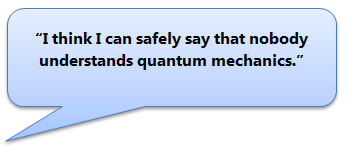Find the force(s) on an object.

Use Newton's 2nd Law to obtain the object's acceleration.

Use the kinematic equations to track the object's motion over time.

Find the force(s) on an object.

We shall now turn our attention to the first step in the standard dynamics general strategy. We shall focus on working out the forces acting on an object, with the understanding that they won't always be provided to us. (So far, I've always given them to you.)

gravitational force

Electromagnetic force

Electromagnetic force

gravitational force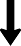If you'd like an accessible introduction to Quantum Mechanics, there are numerous popular books available, such as this one.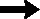This one is easy.

Isaac Newton worked out the model in the late 1600's. It did not originate in a flash of insight but rather was painstakingly pieced together over decades, beginning when Isaac was just 24 years of age. The journey to a complete theory was hampered by a lack of suitable vocabulary, imprecision of data, and shortcomings in the current state of mathematics. Other scientists (such as Robert Hooke) worked on the problem as well, and some were close to working it out.

A key insight for Newton was realizing that "gravity", which pulled apples from trees, extended beyond the confines of the Earth. Once he realized that the Earth's gravity might extend to the Moon, and hold the Moon in its orbit, the rest began to fall into place.

For example, Newton realized that the Moon was constantly accelerating toward the Earth - constantly falling toward the Earth, if you will - just as an apple accelerates toward the Earth when it breaks free of its branch. This implied a force on the Moon, and Newton realized this force could be the same as that which pulls down an apple. The Moon, however, being much farther away, could be expected to feel a much weaker gravitational pull toward the Earth. And, indeed, if Newton was to assume that the force or pull decreased as the inverse square of the distance between the Earth and whatever was falling toward it, the calculated acceleration of the Moon seemed to agree fairly closely with what it was thought to be, obtained from other techniques.

Another key insight - a mind-blowing one! - was that not only is the Moon pulled toward the Earth, and the Earth toward the Sun, but every body pulls on every other body. The Moon pulls back on the Earth, and the Earth pull back on the Sun. Indeed, there is a gravitational attraction, albeit very weak, between you and your bed, a pencil in London and a Coke can in Cape Town, the phone in your pocket and the Curiosity rover on Mars, and every other two items you can imagine (and not imagine), anywhere in the universe. And for this reason, we call this model of gravity Newton's law of universal gravitation.Here is the model. Here is Newton's law of universal gravitation.

The force of gravity (F) between two objects depends on their masses (m1 and m2) and the distance (d) between them.

G is a constant, called the "gravitational constant", which acts as a built-in unit converter. Without G, m1m2 / d2 gives you a force, but it's not in newtons (the SI unit for force). With G, the force comes out in newtons (as long as mass is in kilograms and distance in meters).

Newton himself did not use "newtons" as the unit for force. They had yet to be invented. Nor did he write out this equation using variables; rather, he expressed it in words (in his . He did not speak of G, which was added to the mix later by physicists seeking to standardize units.

Actually, G was not measured until 1798 by the British scientist Henry Cavendish, some 72 years after Newton's death. (The Cavendish experiment, as it is called, is fascinating and worth a Google search.)

This one is not so easy. It is difficult to work out this force directly, yet it is possible to work it out indirectly in particular situations. I have more to say about this force, but we'll save it for later.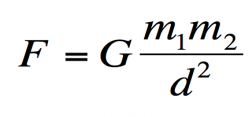Let's use the model to find the force of gravity pulling the Earth and Moon together. (Both the Earth and Moon feel this force.)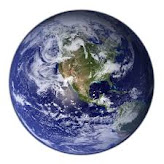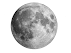mass of Moon = 7.348 x 1022 kg

distance between Earth and Moon = 3.844 x 108 m

(measured from center to center)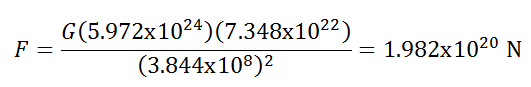mass of Earth = 5.972 x 1024 kg

with G = 6.67408x10-11 m3/(kg·s2),

The Earth pulls on the Moon with a force of 1.98x1020 newtons, and the Moon pulls back on the Earth with the same force. The directions of the forces are toward one another, along the line connecting their centers.

Now, using Newton's 2nd Law: a = F / m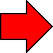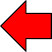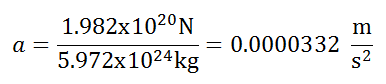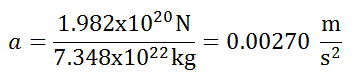If the Moon were the only body to attract the Earth, then the Earth would accelerate toward the Moon at 3.32x10-5 m/s2. Likewise, if the Earth were the only body to attract the Moon, then the Moon would accelerate toward the Earth at 2.70x10-3 m/s2. In reality, other bodies (such as the Sun) pull on both the Earth and Moon, affecting their accelerations. The true accelerations must be calculated from the net forces.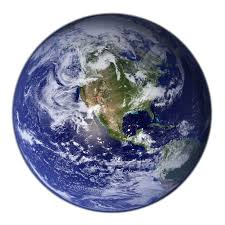Let's calculate the force between an apple and the Earth.

(You know what the acceleration of the apple is going to be, right?)

The mass of the Earth is provided, as is an approximate mass of an apple.

The distance between their centers is the radius of the Earth + a couple of meters.

The Earth is not a perfect sphere, but it has an average radius of 6,371,000 meters.

The addition of a couple of meters is insignificant. Thus, for all objects near the surface of Earth, the distance between the object's center and the Earth's center is taken to be 6.371 x 106 meters.

Newton's Law of Universal Gravitation yields:

Both Earth and the apple feel this tug.

For the apple, Newton's 2nd Law of Motion yields:

a = F / m = 0.982 N / 0.10 kg = 9.82 m/s2   (toward the center of the Earth)

So, how is it that ALL freely falling objects on Earth accelerate at 9.8 m/s2 downward? Can you figure it out before I show you?

mass of Earth = 5.972 x 1024 kg

radius of Earth = 6.371 x 106 mmass of apple = 0.10 kg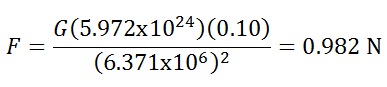We've known for quite some time (via experimentation) that objects near the surface of Earth accelerate downward at about 9.8 m/s2. Now, using Newton's law, we can justify it!Caleb Charland, a Portland, Maine-based experimenter-photographer, wired 300 living apples to an LED. The apples produced just enough current to generate this incredible long-exposure photograph.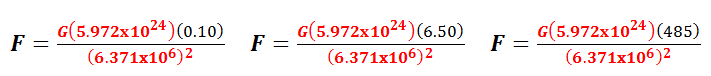It's time to answer the question, Why do all freely falling objects on Earth fall at the same rate?

Take a look at the three calculations below. In each case, I'm finding the gravitational force between some object and the Earth. The only difference between the calculations is the mass of the object. The part in red never changes.

If we think back to our general strategy in dynamics, we calculated this force so that we'd have something to substitute into Newton's 2nd Law, a = F / m or, if you'd like, F = ma.

For our first example, substituting our known force into F = ma yields:

The object's mass (here, 0.10 kg) appears on both sides of the equation, and it can be cancelled out. The part in red, which will always be the same as long as we're near the Earth's surface, must therefore equal the object's acceleration. This will be true for all objects, regardless of mass.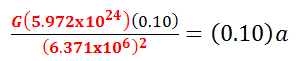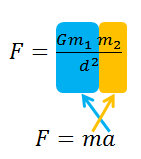You should know what Gm1 / d2 should come out to, when m1 is the mass of the Earth and d is its radius. ("d" is distance, not diameter; be careful!)

Let's calculate it just to be certain: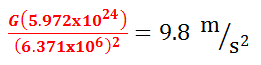And so, yes, as long as something is being gravitationally pulled toward Earth, and as long as it is "near" the surface of the Earth, it will accelerate toward the Earth's center at 9.8 m/s2.

Is the Moon near the Earth's surface? Certainly not! And it accelerates toward the Earth at only 0.00270 m/s2. As we rise from the Earth's surface, the rate of downward acceleration decreases, little by little, (because "d" is growing larger) until it effectively vanishes at great distances from the Earth.

And would an apple dropped from the hand of an astronaut, standing on the Moon, fall downward toward the Moon at 9.8 m/s2? Certainly not! But you could calculate its acceleration given the mass and radius of the Moon.

Actually, do it! The mass of the moon is 7.348 x 1022 kg, and its radius is 1.737 x 106 m.

I'm now in a position to distinguish between mass and weight.

So let's do that!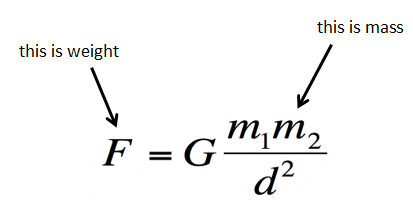Mass is an intrinsic property possessed by an object. All material objects have mass, and that mass is dependent on the size of the object and what it is made of. And the mass of an object cannot be changed by carrying the object to the Moon or Mars or by placing it at the bottom of a deep well.

Weight, on the other hand, is a force. (Specifically, the gravitational force towards a planet, moon or other large body.) An object's weight depends not only on its mass but also the mass (m1) of the other object (the planet or moon) and the distance (d) to the center of that other object. An object's weight, therefore, depends on its location.

A 2.0-kg burrito (!) weighs 19.6 N on Earth but only 3.3 N on the Moon. But it's mass never changes, unless you take a bite of it.

How much do you weigh?

Well, how hard is the Earth pulling on you?

Okay, language can cause a great deal of confusion when words mean different things to different people. "Weight" is such a word. To a scientist, weight is a force, often measured in newtons. To an average American, weight is ... well, how much something weighs, often measured in pounds. Except pounds are NOT the US customary unit for force, as you might guess. Pounds (technically, avoirdupois pounds), as they are typically used by Americans, are a measure of mass. If you weigh 140 pounds in your bathroom, then you will weigh 140 pounds on the Moon, as well. That is your mass, despite the misleading name. You don't know your force-weight. And I don't know mine. But, given that mass and weight are proportional (weight is always 9.8 times larger), it doesn't really matter for most purposes. A person with twice the mass of you will feel twice the gravitational pull and have twice the weight of you, as well. Most Europeans measure their "weight" in kilograms, which is certainly a measure of mass. Although it is still common in Britain to measure "weight" in stones and pounds, as in "11 stone 4 pounds", where 1 stone = 14 pounds.

Let's use Newton's law of universal gravitation to become a bit more comfortable with it.What is the weight of a 9.0-kg dog, on Earth?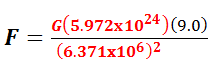9.8

= 88.2 N

What is the weight of a 77.0-kg man, on Earth?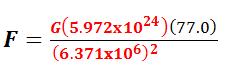9.8

= 754.6 N

What is the weight of a 5000-kg elephant, on Earth?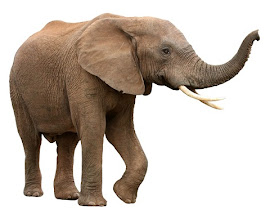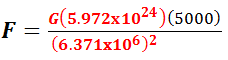= 49,000 N

9.8

What is the weight of a 9.0-kg dog, on the Moon?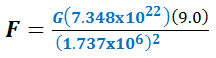1.63

= 14.67 N

What is the weight of a 74.0-kg man, on the Moon?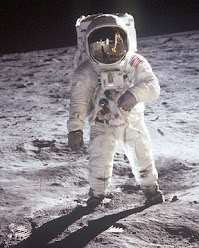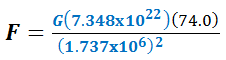1.63

= 120.62 N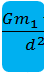is abbreviated as "g"

Thus, on Earth, g = 9.8 m/s2.

We refer to "g" as the acceleration of gravity.

g = 9.8 m/s2

g = 1.63 m/s2

What is the weight of a 74.0-kg man, on a spacewalk?

Buzz Aldrin

1969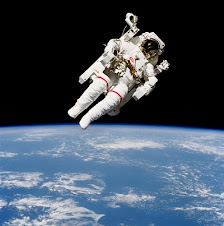This spacewalk is taking place about 200 miles (or 320 km) above the Earth's surface, putting the astronaut at about 6.691 x 106 m from the center of the Earth.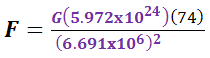8.9

= 658.6 N

Now WAIT a MINUTE!

I thought things in space were weightless.Is the astronaut weightless or is his weight 658.6 newtons?

Both, which goes to show that most people don't know what weightless actually means.

To be weightless is NOT to have a weight of zero. (It would be nearly impossible to have a weight of zero. As you move farther and farther from the Earth, your Earth-based weight approaches zero but never actually gets there. Furthermore, you would have a weight associated with other planets and moons.)

To be weightless is to be FALLING.

Yes, that's right. Read it again. To be weightless is to be falling.

And this astronaut certainly IS falling ... at 8.9 m/s2 toward the Earth.

Not only is HE falling at 8.9 m/s2 toward the Earth, but so is the nearby Space Shuttle and everything within it.

This astronaut currently weighs about 91% of what he does while standing on Earth.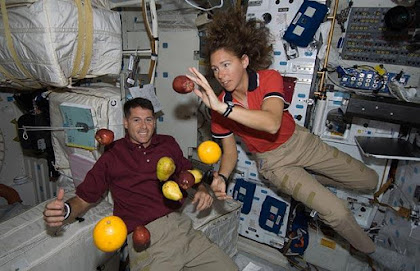All of this stuff --

the people, the fruit, the books, the actual shuttle --

are falling toward the Earth at 8.9 m/s2.

To fall AROUND something is to orbit.

To fall INTO something is to crash.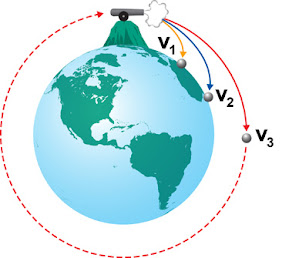This illustration is meant to show how an object can "fall" around the Earth. If a cannonball is going fast enough, the rate at which it falls could match the rate at which the Earth curves away.

How fast must something be going to fall around the Earth?

This is how fast the space shuttle (and that fruit) is going.Weightless for all of one second.

Before we move on,

we have to calculate the gravitational force between two small objects. Remember, every two pair of objects in the universe exert an attractive force on one another. As you'll see from the calculation below, the force is extremely small. It's so small that we ignore it; we forget it exists. But it does exist!mass of truck = 2722 kg

mass of man = 73 kg

distance between them = 10 m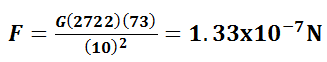That's right, both man and truck experience a force of just 0.000000133 N. Were there no other forces acting on the man, he'd accelerate toward the truck at 0.00000000182 m/s2.

But of course, nearby people and trees attract him in every other direction. Tiny forces pull him in every which way, effectively canceling out, producing no acceleration. The only gravitational force worth mentioning is the one holding him to the Earth.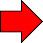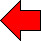gravitational force

We've covered the Gravitational Force in some depth, now.

You should be comfortable calculating the gravitational force between any two pair of objects, you should know the meaning of "g", you should understand the difference between mass and weight, and you should know what it means to be weightless.

As we move forward, we'll have to discuss strategies for determining the Electromagnetic Forces that act on the objects around us.

Recall, we are now adhering to the dynamics general strategy.

Practice problems

For reference:

G = 6.67408x10-11 m3/(kg·s2)

For reference:

G = 6.67408x10-11 m3/(kg·s2)

Forces

Kinematics